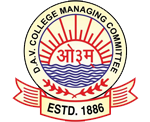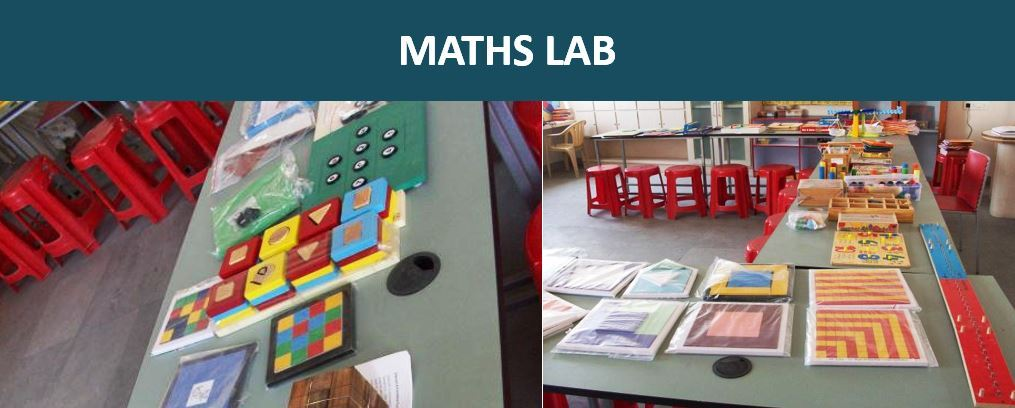CBSE Affiliation No: 430183
Latest News

Math LabThe school owns a well equipped Maths lab which is utilized by the Mathematics teachers. We all understand that maths cannot be learnt. It has to be understood and practiced. The Maths lab takes this concept a little further. Here students are made to experience the various principles and fundamentals of mathematics. In Maths lab, students work together and learn Maths in a fun way method. Maths, measurement and relationships between various principles are not left to some strange imagination, they are clarified by actual demonstration. This makes maths more real and hence better understood.